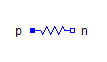HBridge - MapleSim Help

HBridgeDescription The H-bridge component is a four-quadrant DC/DC converter. It consists of two single-phase DC/AC converters which are independently controlled.If the firing inputs ${\mathrm{fire}}_{p}$ and ${\mathrm{fire}}_{n}$ are inverses, the two legs are controlled symmetrically so that full positive or negative output voltage can be generated. To see an example model that uses this component, search for HBridge in the MapleSim search bar.Connections

 Name Description Modelica ID $\mathrm{heatPort}$ Conditional heat port heatPort $\mathrm{enable}$ Boolean input; enable ${\mathrm{fire}}_{p}$ and ${\mathrm{fire}}_{n}$ inputs enable ${\mathrm{fire}}_{p}$ Boolean input; firing signal of positive potential transistor fire_p ${\mathrm{fire}}_{n}$ Boolean input; firing signal of negative potential transistor fire_n ${\mathrm{dc}}_{\mathrm{p1}}$ Positive DC input dc_p1 ${\mathrm{dc}}_{\mathrm{n1}}$ Negative DC input dc_n1 ${\mathrm{dc}}_{\mathrm{n2}}$ Negative DC output dc_n2 ${\mathrm{dc}}_{\mathrm{p2}}$ Positive DC output dc_p2ParametersGeneral Parameters

 Name Default Units Description Modelica ID ${R}_{\mathrm{on}\left(\mathrm{tran}\right)}$ $1·{10}^{-5}$ $\mathrm{\Omega }$ Transistor closed resistance RonTransistor ${G}_{\mathrm{off}\left(\mathrm{tran}\right)}$ $1·{10}^{-5}$ $S$ Transistor opened conductance GoffTransistor ${V}_{\mathrm{knee}\left(\mathrm{tran}\right)}$ $0$ $V$ Transistor threshold voltage VkneeTransistor ${R}_{\mathrm{on}\left(\mathrm{diode}\right)}$ $1·{10}^{-5}$ $\mathrm{\Omega }$ Diode closed resistance RonDiode ${G}_{\mathrm{off}\left(\mathrm{diode}\right)}$ $1·{10}^{-5}$ $S$ Diode opened conductance GoffDiode ${V}_{\mathrm{knee}\left(\mathrm{diode}\right)}$ $0$ $V$ Diode threshold voltage VkneeDiode Use Heat Port $\mathrm{false}$ True (checked) means heat port is enabled useHeatPortEnable Parameters

 Name Default Units Description Modelica ID Use Constant Enable $\mathrm{true}$ True (checked) means disable Enable port. useConstantEnable Constant Enable $\mathrm{true}$ True (checked) means firing signals are always enabled. constantEnableModelica Standard Library The component described in this topic is from the Modelica Standard Library. To view the original documentation, which includes author and copyright information, click here.